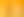How Big?

# 5. How Big is That Balloon?Suggested Learning Intentions

• To compare different lengths, masses, capacities, and temperatures
• To convert between a range of units
• To theorise the volume of a sphere

Sample Success Criteria

• I can compare different measurements
• I can convert between different units of measure
• I can calculate the volume of a sphere
• I can use manipulatives to model and demonstrate my thinking
• Supply of balloons (at least the 30cm diameter variety)
• Flexible measuring tape or lengths of cotton
• Class set of 30cm rulers
• A few empty one-litre containers such as milk cartons or soft drink bottles
• Bucket of water

Provide students with a range of pre-made sorting cards (available in the Materials and texts section above). Each set of cards shows a range of measures.

Have students compare each set of cards and arrange the measurements in order from smallest to largest. To order the cards, students must perform several conversions.

Provide cards to support the wide range of abilities in the classroom. You may need to create a range of conversion cards to ensure all students can engage with this activity. When creating additional conversion cards consider the units which your class has been experiencing confusion with. Confusion creates an ideal teaching moment; it also allows you to assess their understanding of decimal numbers.

Enable students by creating sets of cards which compare measurements of length or weight. Some students might benefit from comparing measurements that they are most familiar, or measurements which they can physically compare. For example, a range of lengths they can measure out in the classroom.

Extend students by creating sets of cards which compare measurements of area, volume, and capacity. Have students compare units from the metric system with imperial units. Converting units in the metric system is a much simpler process than converting and comparing between the metric and imperial system. Therefore, it is not recommended that you provide students with these activities until they have a solid understanding of converting within the metric system.

The Two-Litre Balloon problem is based on an activity from Maths300 lesson and is used here with permission. This activity supports students to develop their understanding of volume.

Begin by asking students to take one balloon each and blow it up until they estimate it reaches a volume which is double that of a 1 litre bottle. Have a variety of one litre bottles on hand for student reference. Once they have blown up their balloon, students use a range of problem-solving strategies to measure the balloon, to determine if the balloon’s volume is close to 2 litres.

Encourage students to use their knowledge of circles to determine the volume of the balloon.

Students develop a method of their own to determine the volume of the balloon. At the conclusion of the activity, ask the students to reflect on their problem-solving strategies and suggest ways in which they could improve their methodology next time. Discuss whether this shape of the balloon impacts on the accuracy of their estimate. For example, would a cylindrical balloon make it easier to estimate the volume?

Enable students by providing them with a bucket of water. Demonstrate how to submerse the balloon in the bucket of water and measure the amount of water displaced to calculate the volume of the balloon. Support students to submerse their own balloons and to measure the level of water displaced. You could read "Mr Archimedes' Bath" by Pamela Allen to students to reinforce the concept of water displacement and volume.Once a student has calculated the dimensions of a 2-litre balloon extend them by asking them to calculate the dimensions of a 3-litre balloon. Some students will automatically assume that the diameter increases by 50%.Ask students to record the strategies they used to perform the conversions in the Get started section of this stage.

Were there any units they were unfamiliar with?

Were there any units where they became confused? What did they do to stop themself from being confused?

Did they find it easier to convert to an intermediate unit rather than go from one unit to another (for example millimetres to kilometres)

Enable students by asking them to write a set of step-by-step instructions to convert from one unit to another for another student to follow. Encourage them to provide examples to add clarity to their instructions.

Extend students by selecting the conversions you ask them to write instructions for. Depending on the current knowledge and understanding of your students, use a range of units and include decimal and fractional numbers.

Australian Association of Mathematics Teachers, n.d. Maths 300. [Online]
Available at: https://maths300.com/lessons/MTk2TWF0aHNMZXNzb24zMDA=
[Accessed 15 March 2022; Note: access to this resource requires a maths300 subscription].